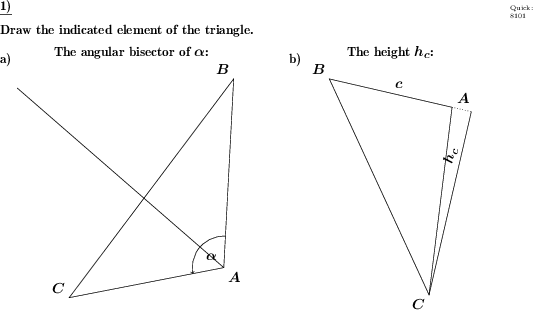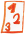Custom math worksheets at your fingertips# Details for problem "Triangle: Draw heights, medians and bisectors"

Quickname: 8101

## Summary

Draw heights, medians, angle bisectors or perpendicular bisectors for a triangle.

## Examples## Description

A triangle is given.

Depending on the problem statement, a specific

- perpendicular bisector

- height

- median

or

- angle bisector

is to be drawn.

The number of problems is configurable. For every type of geometric line, it can be defined whether it can be asked for.

Download free worksheets for this math problem here. The worksheet contains the problems only, the solutions sheet includes the answers. Just click on the respective link.

•Worksheet 1Solution sheet with answers
•Worksheet 2Solution sheet with answers
•Worksheet 3Solution sheet with answers

If you can not see the solution sheets for download, they may be filtered out by an ad blocker that you may have installed. If this is the case, please allow ads for this page and reload the page. The solution sheets will then reappear.

• Do the sample worksheets do not really fit?
• Do you need more math worksheets, with a different level of difficulty?
• Would you like to combine different problems on a worksheet and adjust them to your needs?
• As a teacher, you can put together your own worksheets using the automatically generated math problems provided.
With a free initial credit, you can start creating your own math worksheets in a few minutes.

It does not cost anything to try! Register here, to create custom worksheets now!

## Customization options for this problem

Parameter
Possible values
Number of problems
1, 2
Yes, No
Yes, No
Yes, No
Yes, No

## Similar problems

Remark
Description
basic view on angle bisectors
Construct the bisector for a given angle using just a compass and a straightedge.
perpendicular bisectors in a triangle, in conjunction with the circumcircle
The circumcircle and perpendicular bisectors have to be drawn for a given triangle.
angle bisectors only, in conjunction with the incircle
The incircle and angle bisectors have to be drawn for a given triangle.
medians in a triangle, in conjunction with the centroid
The medians and centroid have to be drawn for a given triangle.

Deutsche Version dieser Aufgabe
These informational pages with samples describe math problems that can be combined on custom math worksheets with solutions for home and school use.